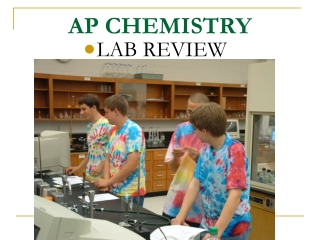DownloadDownload PresentationAP CHEMISTRY

# AP CHEMISTRY

Télécharger la présentation## AP CHEMISTRY

- - - - - - - - - - - - - - - - - - - - - - - - - - - E N D - - - - - - - - - - - - - - - - - - - - - - - - - - -
##### Presentation Transcript

1. AP CHEMISTRY • LAB REVIEW

2. Exp 1 • Zn + 2HCl  ZnCl2 + H2 • Cu + HCl  NO Reaction • Activity Series

3. Exp 3 • Empirical Formula • ____g Cu / 63.5 = mol • ____g I / 126.9 = mol • Then divide by the smaller. • Iodine makes violet vapors

4. EXP 4a % Composition • 2KClO3(s) 2KCl(s) + 3O2(g) • g of O2 lost x 100 =%O2 • g of KClO3 started

5. EXP 6a Hydrates • 1.28g CuSO4 /159.6 g/mol = 0.00802 mol • 0.72g H2O / 18.0 g/mol = 0.0400 mol • 0.00802 mol / 0.00802 mol = 1 • 0.0400 mol / 0.00802 mol = 5CuSO4.5H2O • Copper(II) sulfate pentahydrate

6. hydrated anhydrous

7. EXP 6 % YIELD • Cu + 4HNO3 Cu(NO3)2+2NO2 +2H2O • Cu + 4H+ + 2NO3-  Cu2+ + 2NO2 + 2H2O p11

8. Exp 6 • Zn + CuSO4 ZnSO4 + Cu • Blue Clear • %yield =exp/calc x 100

9. EXP 9 Rates of Evaporations • IONIC • ION-ION • COVALENT • NONPOLAR-LDF • POLAR – DIPOLE-DIPOLE and HYDROGEN BONDING • Evaporation is a cooling process!

10. EXP 12HESS’S LAW

11. EXP 14 • ACTIVITY SERIES • USE THE STANDARD REDUCTION POTENTIALS

12. EXP 15FREEZING-POINT DEPRESSION Temp Time

13. EXP 15 • ΔT = Kf x m • MW = g1 x 1000g/kg g2 x m g1 = solute g2 = solvent m = molality

14. EXP 17KINETICS • 0TH order [conc] Time

15. EXP 17 • 1st order ln [conc] Time

16. EXP 17 • 2nd order 1/[CONC] Time

17. Exp 21Conductivity from Chem I

18. EXP 21 • GOOD electrolytes • Strong acids and bases • Salts dissolved in water (aqueous) • Molten salts • Metals • Ions must be able to flow.

19. EXP 21 • Poor or Weak electrolytes • Weak acids and bases • Tap water

20. EXP 21 • NDC • DISTILLED WATER • SUGAR WATER • COVALENT COMPOUNDS • Solid salts

21. EXP 21Ka of a WEAK ACID • pH = pKa at ½ of the equivalence point pH 7- ml of NaOH added

22. EXP 22aShapes of Molecules • Know the VSEPR Theory card • Know polarity • Know hybrid orbitals • Bond angles

23. EXP 23Titration Curves • Strong acid vs strong base pH 7- ml of NaOH added

24. EXP 23 • STRONG ACID vs WEAK BASE pH 7- ml of NH4OH added

25. EXP 23 • Weak Acid vs Weak Base pH 7- ml of NH4OH added

26. EXP 23 • H3PO4 vs NaOH pH 7- ml of NaOH added

27. EXP 24GRAHAM’S LAW • NH3(g) + HCl(g) NH4Cl(s) HCl NH3

28. EXP 25 • Heat of Neutralization • q = m Cp ΔT • Then divide by the # of moles of water produced. Measured in kJ/mol

29. EXP 26KINETICS • [S2O82-] [I-] Rate (M/s) • 0.10M 0.10M 5 • 0.10M 0.20M 10 • 0.20M 0.10M 10 Rate = k[S2O82-]1[I-]1

30. EXP 29 • P1V1/T1 = P2V2/T2 • Subtract out the vapor pressure (Dalton’s Law) • . . • (collected over water) • 1 mole any gas = 22.4 liter (@ STP) Press inside = press outside only if water levels =.

31. EXP 30MW of a Vapor • MW = mRT PV

32. EXP 31 (from Chem I)Solubility of KNO3 • Solute + solvent + heat  solution • Endothermic if increase solubility with increasing temperature. g KNO3/100g of water Temperature oC

33. EXP 33 Spec 20 • A = abcAbs & conc direct prop. • λ is set for the complimentary color of the solution. • Absorbance = 2 – log(%T) • Solution must have a color.

34. EXP 37 • ISOMERS

35. Structural isomers • n-pentane • 2-methyl butane • 2,2- • dimethylpropane

36. Optical isomers

37. Geometric isomers (cis-trans)

38. Functional isomers

39. EXP 41HYDROLYSIS • Salt + water  acid + base

40. EXP 42ACID-BASE TITRATION • ACID + BASE  SALT + WATER • (#H)M1V1 = M2V2(#OH) • Indicator is phenolphthalein • KHP is a standard

41. EXP 43EQUATIONS • SOLUBILITY RULES • YELLOW PPT • PbI2 • PbCrO4

42. EXP 44RED-OX TITRATION • FeSO4 + KMnO4 + H2SO4 Fe2(SO4)3 + MnSO4 + K2SO4 + H2O

43. Exp 13Flame Test (from Chem I) • Na yellow • K lavender • Ca & Li & Sr red • Ba & Cu & B green

44. Na+ K+ Sr2+ Ba2+ Cu2+ Se